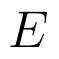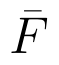Select Page

# CBSE Maths 12 Science Inverse Trigonometric Functions MCQ Answers in English

CBSE Maths 12 Science Inverse Trigonometric Functions MCQ Answers in English to enable students to get Answers in a narrative video format for the specific question.

Expert Teacher provides CBSE Maths 12 Science Inverse Trigonometric Functions MCQ Answers through Video Answers in English language. This video solution will be useful for students to understand how to write an answer in exam in order to score more marks. This teacher uses a narrative style for a question from Inverse Trigonometric Functions not only to explain the proper method of answering question, but deriving right answer too.

Please find the question below and view the Answer in a narrative video format.

Question:

## Similar Questions from CBSE, 12th Science, Maths, Inverse Trigonometric Functions

Question 1 :is equal to: (View Answer Video)

Question 2 : Iffind x. (View Answer Video)

Question 3 :is equal to : (View Answer Video)

Question 4 :Then x is equal to : (View Answer Video)

Question 5 :is equal to : (View Answer Video)

### Linear Programming

Question 1 : The objective function is maximum or minimum, which lies on the boundary of the feasible region. (View Answer Video)

### Probability

Question 1 : A die is thrown 6 times. If 'getting an odd number' is a 'success', what is the probability of obtaining:
exactly 5 successes?

Question 2 :  Four cards are drawn successively with replacement from a well shuffled deck of 52 cards. What is the probability that:

Question 3 : A couple has 2 children. Find the probability that both are boys, if it is known that one of them is a boy.  (View Answer Video)

Question 4 : Out of a group of 30 honest people, 20 always speak the truth. Two persons are selected at random from the group. Find the probability distribution of the number of selected persons who speak the truth. Also find the mean of the distribution. What values are described in this question?     (View Answer Video)

Question 5 :  If E and F are independent events, then show thatandQuestion 1 : Evaluate :(View Answer Video)
Question 2 : Find the integral of the function. (View Answer Video)
Question 3 : Find :(View Answer Video)
Question 4 : Evaluate :(View Answer Video)
Question 5 :(View Answer Video)